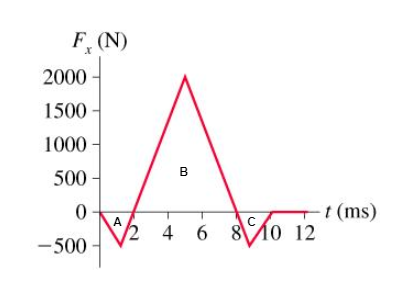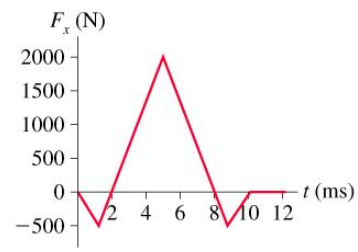# Problem: What is the impulse on a 3.0 kg particle that experiences the force described by the graph in the figure?

###### FREE Expert Solution

Impulse is equal to the area under the curve. We'll sum the area enclosed by the three triangles.

Area of traingle,

$\overline{){\mathbf{A}}{\mathbf{r}}{\mathbf{e}}{\mathbf{a}}{\mathbf{=}}\frac{\mathbf{1}}{\mathbf{2}}{\mathbf{×}}{\mathbf{b}}{\mathbf{a}}{\mathbf{s}}{\mathbf{e}}{\mathbf{×}}{\mathbf{h}}{\mathbf{e}}{\mathbf{i}}{\mathbf{g}}{\mathbf{h}}{\mathbf{t}}}$For triangle A,

Base, b = (2 ms - 0 ms) = 2 × 10-3 s

Height, h = - 500 N

For triangle B,

Base, b = (8 ms - 2 ms) = 6 × 10-3 s

Height, h = 2000 N

90% (82 ratings)###### Problem Details

What is the impulse on a 3.0 kg particle that experiences the force described by the graph in the figure?